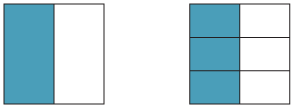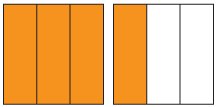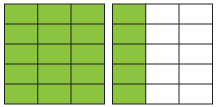### Using squares

We can use squares to see that the area representing $$\dfrac{1}{2}$$ equals the area representing $$\dfrac{3}{6}$$.#### Equivalent improper fractions

We can use squares to show that $$\dfrac{4}{3}$$ is equivalent to $$\dfrac{20}{15}$$. The area of each large square is 1.

1.2.Shaded area A represents the fraction $$\dfrac{4}{3}$$ and shaded area B represents the fraction $$\dfrac{20}{15}$$. The two areas are the same, so $$\dfrac{4}{3}$$ is equivalent to $$\dfrac{20}{15}$$.